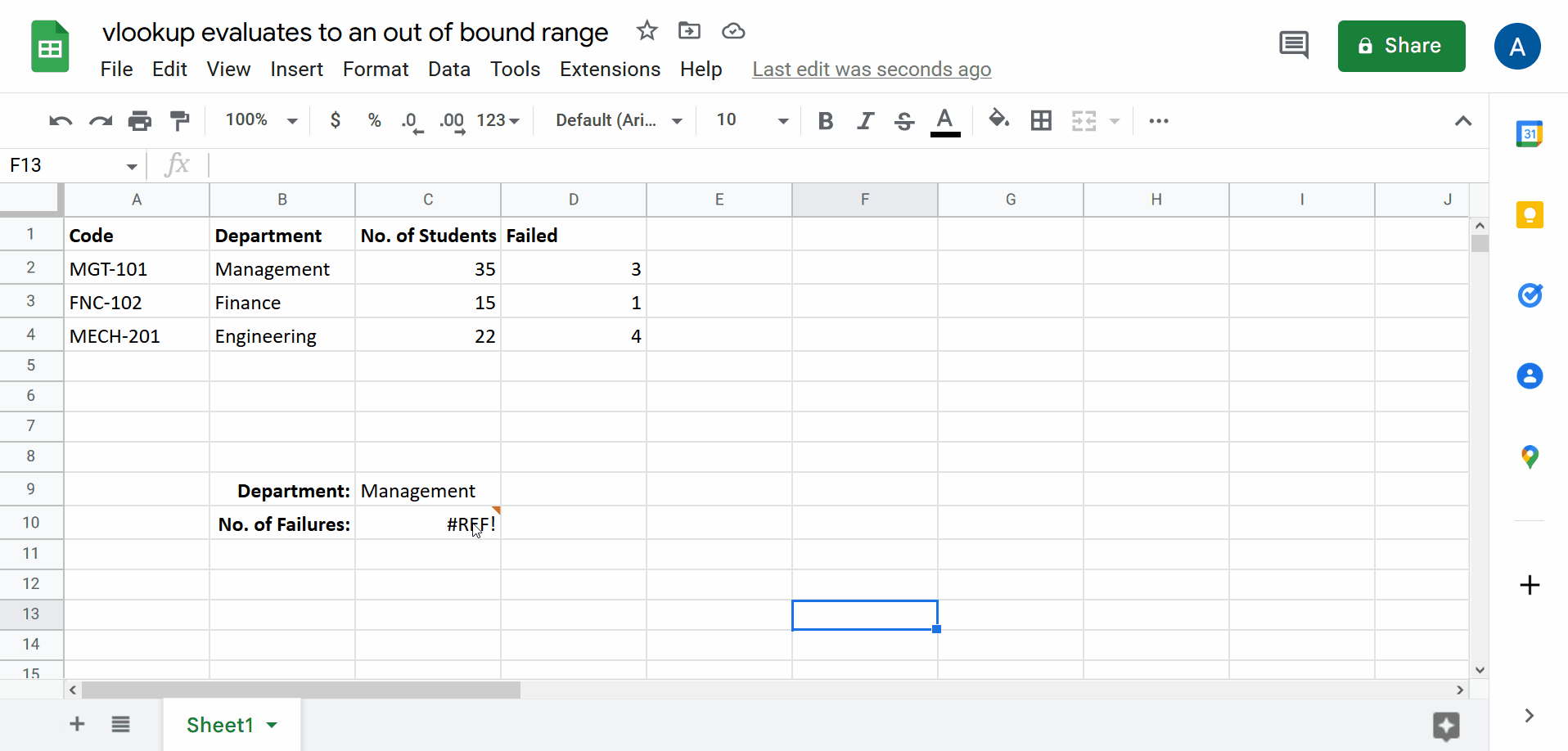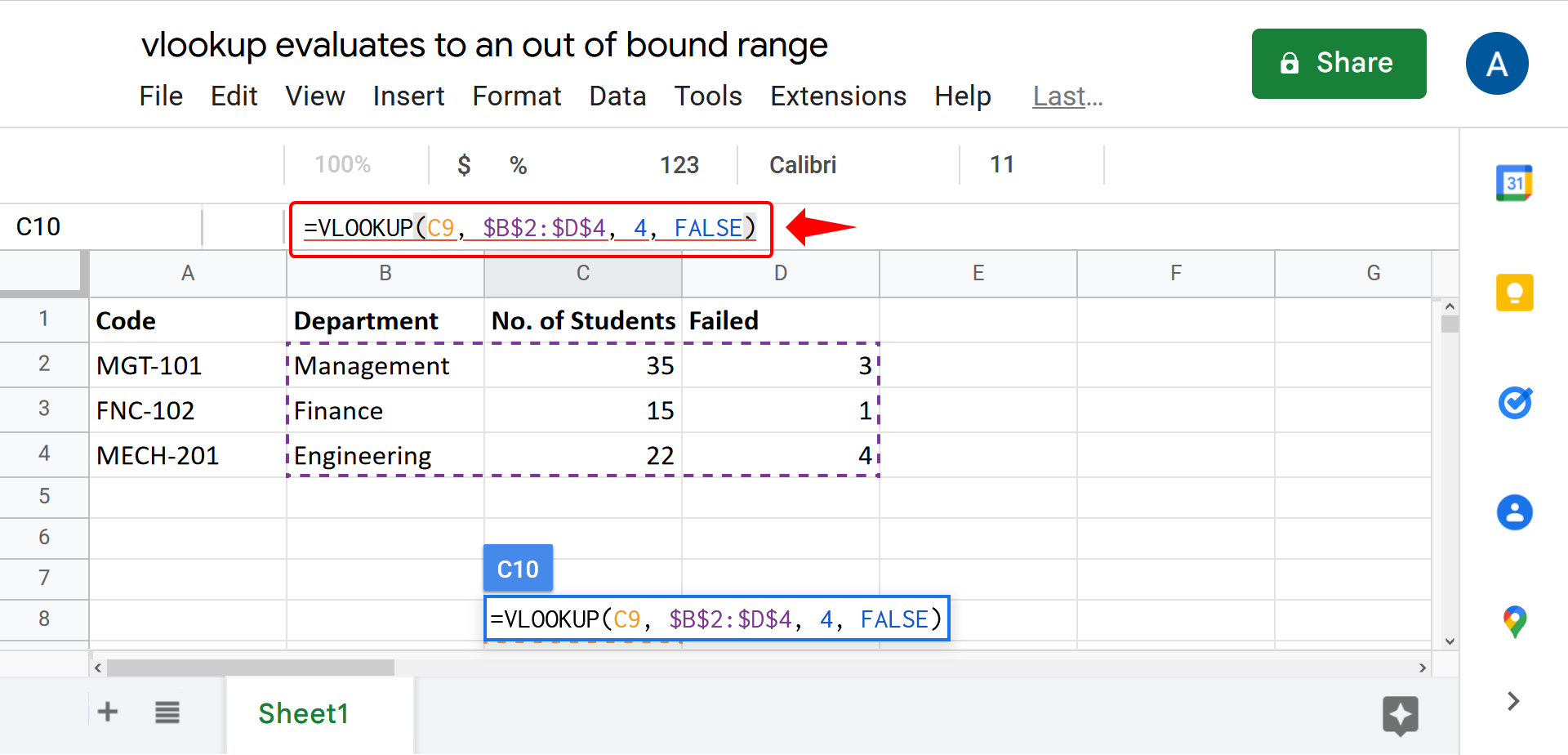# Why Vlookup evaluates to an out-of-bound range in Google Sheets(+ diagnosis)

While working in google sheets one might encounter an error that Vlookup evaluates to an out of bound range as shown in the image above.

So let’s see how the problem can be resolved.VLOOKUP is a very handy function that allows looking up values in a column and picking corresponding values from another column within a row.

### Step 1 – Understanding the formula– The formula for VLOOKUP is shown below.

=VLOOKUP(search_key, range, index, [is_sorted])

– The first attribute is the search_key and is the value that we want to look up. In our current scenario it is the department name that is mentioned in the cell C9.

– The second is the range. The range refers to the rows and columns from which this formula is going to pick values. In our case it is the range from cell B2 to cell D4.

– The third attribute is the index of the column we want to get the value from. The column that we want to get value from is the Failed column.

– And the final attribute is the optional attribute [is_sorted]. This attribute tells the system if the values in the column are sorted in which case the closest match will be returned.

– The formula provided for our case is shown in the image below.

### Step 2 – Removing the error– In the formula we need to provide the index of the column as the third attribute with respect to the range in the second attribute.

– We see that the range spans 3 columns. However, the index attribute is being passed the value 4 which is wrong. We need to get the value from the Failed column which is the 3rd column. 4 is, therefore, out of bound and hence the error.

– So we are going to replace the value 4 with 3 and press enter.

– And now we have the formula implemented without error.